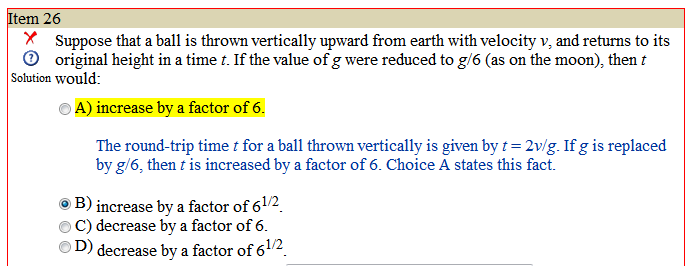Premed 101 Forums

# Uniformly Accelerated Motion Problem

## Recommended PostsThe way I solved it was saying:

d= Vot + 1/2(a)(t^2)

t in the question refers to the total time of the trip. Since the trip up and down is the same amount of time, whatever happens to half the trip will also happen to the full thing. I then said, Vo = 0, because at the top of the flight you would have no velocity. This lead me to saying:

d = (1/2)(a)(t^2)

Which can be rearranged to:

sq root (2d/a) = t

Now suppose a = g/6 as suggested in the question, then the factor that t is changing by is sq root 6.

This was no the right answer. I don't know what I did wrong. This is a pretty simple problem, so I'm hoping I don't get something like this wrong again! Any help would be super appreciated. I don't understand how they got to t = 2v/g, and why my method failed.

##### Share on other sites

Okay, this is how they got their answer:

Since the ball goes up and then goes down to the exact same location, d=0 (d is the total distance traveled). So you get

0 = v0*t + 1/2(a*t^2)

--> -v0*t = 1/2(a*t^2)

--> -v0 = 1/2(a*t)

--> t = -2*v0/a

a = -g (since it's in the opposite direction to the initial motion, and you can't have a negative time so something has to get rid of that negative sign)

--> t = 2*v0/g

I'm still trying to figure out why your way didn't work, and I suspect that it might not be the exact same amount of time to go up as to go down.... I'll think about that a bit and get back to you. :

##### Share on other sites

Ah, thanks very much astrogirl. I could have avoided my problem by saying d is zero (as the displacement in this case is zero). I've been trying to figure out why splitting it into two half-trips wouldn't work though. Since sq root 6 was an answer, they definitely knew some people would fall into the trap I fell into - but I can't seem to realize what this trap is yet!

##### Share on other sites

Ah, thanks very much astrogirl. I could have avoided my problem by saying d is zero (as the displacement in this case is zero). I've been trying to figure out why splitting it into two half-trips wouldn't work though. Since sq root 6 was an answer, they definitely knew some people would fall into the trap I fell into - but I can't seem to realize what this trap is yet!

The reason it doesn't work is because distance-to-the-top changes depending on your acceleration, assuming the same initial velocity.

d1 = distance to top at gravity g

d2 = distance to top at gravity g/6

d1 = vo*t + 1/2*g*t^2

d2 = vo*t + 1/2*(g/6)*t^2

Simple substitution shows that

d2 - d1 = 1/2*(g/6)*t^2 - 1/2*g*t^2

d2 - d1 = -11/24g*t^2

Going to the top, velocity is positive and gravity is negative, therefore (-11/24g*t^2) will be a positive term (the negatives cancel out)... and therefore d2 > d1.

--------------------------------------------

Another way to look at it is simply this: the only constants you are given are v and a (g or g/6). Therefore, it is only appropriate to solve for t based on those constants, which is what astrogirl has done.

I'm still trying to figure out why your way didn't work, and I suspect that it might not be the exact same amount of time to go up as to go down.... I'll think about that a bit and get back to you. :

That is incorrect. In an ideal physics environment, the time to go up always equals the time to come down (unless it's something like throwing it off a cliff, obviously).

##### Share on other sites

Yeah, thanks for posting that estairella! That was really driving me crazy. I spent a while trying to decide whether it should take the same amount of time to go up and come down, which involved actually throwing some stuff up in the air and watching it, and then realized that I could just do some math to figure it out. But I still didn't see why Law's way didn't work, so I'm glad you figured it out.#### Archived

This topic is now archived and is closed to further replies.

×

• Pages